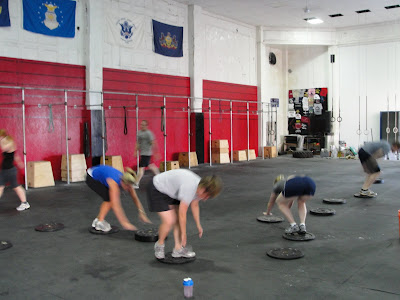## 8.03.2010

FRAN VIDEO

W.O.D. 8.4.10

AMRAP (as many reps as possible) in 12 Minutes of:
Burpee Box Jumps (13"/17")

Immediately after completing the AMRAP complete max reps of Body weight Deadlift in 90 Seconds.

Free Yoga Event:
Salutation Nation is an event Hosted by Lululemon Athletica on Saturday August 7th at 9AM at The Piazza in Northern Liberties- Philadelphia North Second Street and Germantown Avenue, Philadelphia, PA there will be a free yoga practice led by some of our area's most inspiring teachers. This event will be going on world wide, the North American goal will be to gather 25,000!!

Video:
Interview with Chris Spealler on Day 2 at the 2010 CrossFit Games - video [wmv] [mov]

AND

Dave Lipson "Isabel" - video [wmv] [mov]

Quote:
Vision. It reaches beyond the thing that is, into the conception of what can be. Imagination gives you the picture. Vision gives you the impulse to make the picture your own.
-Robert Collier

Results:
Rx +
Mike F: 66+12 (21") = 78
Olan: 93+21 (21") = 114
Plentus: 110+14 (25" 225#) = 124
Sam B: 111+13 (21") = 124
Joe A: 64+13 (25") = 77

Rx
Liz 90+7 = 97
Jen S: 99+11 = 110
Hannah: 60+12 = 72
Patti: 65+10 = 75
Miranda: 86+9 = 95
Josh: 60+24 = 84
Jeff: 83+13 = 96
Jay E: 82+24 = 106
Mel: 93+13 = 106
Jason: 94+25 = 119
Peterson: 75+10 = 85
Ellie: 85+11 = 96
Dorothy: 98+9 = 107
Sam D: 100+11 = 111
Kristen S: 70+10 = 80
Joe M: 65+10 = 75
Brian R: 107+13 = 120
Danny: 85+12 = 97
Jerry: 105+20 = 125
Kevin: 109+16 = 125
Aimee: 102+26 = 128

Scale
Dianne: 20?+22 = 42+
WW: 70+11 = 81
Rachael: 98+18 = 116
Rob Ph: 87+12 = 99
Lindsey: 63+15 = 78
SAZ: 61+7 = 68
Christine: 70+15 = 85
Megan: 78+15 = 93
Bevin: 64+14 = 78
Kim G: 75+17 = 92
Tim H: 30+6 = 36
Tim G: 72+15 = 87
Karen: 37+13 = 50
Holly 42+ 21 = 63
Jen N. 53+13 = 66
Flounder 80+6 = 86
Chris C. 58+14 = 72
Sandy 50+15 = 65
Jason Bo. 62+30 = 92

Photos:Miranda said...

Mel~ can I grab that recipe for Pesto from you?

Melanie said...

I forgot to grab it this morning since I was running late but I can email it to you later tonight when I get home or I can post it on the blog if anyone else wants it.

Patti said...

Great video Chris and I'm not just saying that cause I'm in it.lol

Chris P. said...

nice job 6:30 crew, esp. new comers Jason, Chris, and Sandy!

Sam B. 111/13=124 (165/21") Rx+
Holly 42/21=63 (65/13")
Jen N. 53/13=66 (45/13")
Liz T. 90/7=97 (135/13")
Flounder 80/6=86 (205/13")
Chris C. 58/14=72 (75/17")
Sandy 50/15=65 (45/6")
Jason Bo. 62/30=92 (75/17")

Chris P. said...

sorry, Liz did Rx but I didn't note itJoe A said...

I did 25 inch for the box jumps

Aimee Lyons said...

@Liz & Joe A. - Got em fixed for you! thanks!